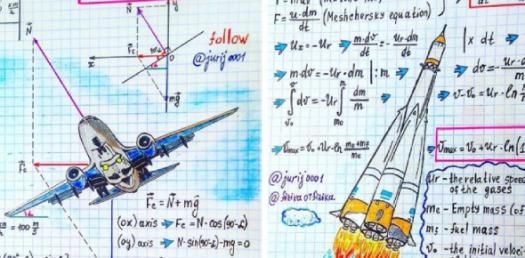# Physics Questions: Knowledge Test! Trivia Quiz

21 QuestionsSettingsPhysics is the science of matter, and understanding it makes us know how to make work easier. In this knowledge test, we get to test out knowledge on different types of measurements and instruments too. Do you know enough about the topic to get the full score? The best way to find out is to take the actual quiz and prove it.

Related Topics
• 1.
A measuring tape is most commonly used by a cloth dealer.
• A.

True

• B.

False

• 2.
Utensils like spoons, glasses, and cups are used to measure capacity in non-standard units.
• A.

True

• B.

False

• 3.
The normal temperature of human body is 37F.
• A.

True

• B.

False

• 4.
Mass of an object tells us how heavy or light that object is
• A.

True

• B.

False

• 5.
A ruler has markings in centimetres and inches.
• A.

True

• B.

False

• 6.
We use any thermometer to measure the temperature of our body.
• A.

True

• B.

False

• 7.
APCITYAC
• 8.
URERL
• 9.
PERATMETURE
• 10.
MAGRS
• 11.
What is the standard unit to measure the length?
• 12.
What is the standard unit to measure capacity?
• 13.
Which quantity tell us how heavy or light an object is
• 14.
Which device is used to measure the temperature of our body?
• 15.
What is the normal temperature of human body?
• 16.
The measurement of amount of liquid that a container can hold
• 17.
The degree of hotness or coldness of a body
• 18.
An object has the amount of matter in its body
• 19.
It is the measurement of an object from end to end
• 20.
Daily temperature is recorded by